Categories
Pages
-Susy edges

August 2nd, 2014 | by

Two weeks ago, the  SUSY conference took place in Manchester, and we already had a nice report from Mathieu and Jory here in our blog. However, I also want to draw your attention to this talk about an overview of CMS searches for supersymmetric particles at the LHC. Generic searches for supersymmetric particles depend mainly on two possible observations: in most supersymmetric scenarios, one has a lightest stable supersymmetric particle (that can play the role of the dark matter candidate). This particle, if produced at a collider like the LHC, does not decay any more (stable!) and does not leave any trace in the detectors. No trace? No!

It carries away a lot of momentum and since we know the momentum balance of the collision (at least in two directions…), such a particle can expose itself through an imbalance in the momentum balance, which is called missing energy. The other observation one looks for are the so called Susy edges, and this is what we want to discuss here.  These edges denote a generic shape in a specific distribution of two standard model particles that come out of a decay of a supersymmetric particle (actually, the decaying particle need not be a supersymmetric one. Any particle that decays in such a way will do the job, with some restrictions). How do you observe such an edge? For this, these heavy supersymmetric particles have to be produced, e.g. like this via a Z Boson: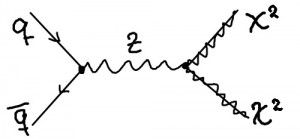In this example, two neutralinosare produced. These are not stable and decay inside the detector via an intermediate slepton (the supersymmetric partner to a lepton)into two leptonsand the lightest neutralinoin a decay chain like this:The only particles which one can measure in the detectors in this case are the two leptons (muons or electrons). So one investigates distributions gained from their energies and momenta. In this case, one builds the invariant mass distribution of the two leptons, that is their four-momenta summed up and is called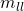. This distribution looks like an “edge”.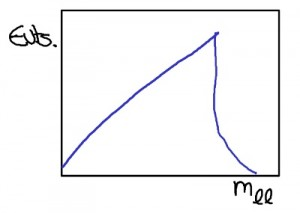Depending on the specific mass constellations of the particles involved in this decay, we can have two type of edges. If the slepton is lighter then the neutralino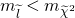, then this distribution gives a sharp edge and looks roughly like the one in the left plot. If the mass of the neutralinois lighter then the slepton, then the edge is not that sharp (right plot).
Experimentalists now search for these kind of shapes in the corresponding distributions to get a hint towards the production and decays of supersymmetric particles.This search which is mentioned in the talk above, looks for leptons with opposite sign and same flavor(that measor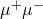). This is one of the plots you can find on the analysis that shows the invariant mass distribution of those leptons. The black points are the data,  the blue line is the fit. The red peak comes from the Drell-Yan-Process, you can basically see the Z-Boson there. The green dotted edge shows the fit of a possible signal to the data. However, as the abstract of the analysis says, this is not statistically significant and probably only a statistical fluctuation. Let’s wait for the full discussion, but I guess no one will bet on this edge.